# Addition and subtraction of fractions

### Addition of fractions with the same denominators

To add two fractions with the same denominators, add their numerators, and the denominators remain unchanged: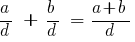### Examples of addition of fractions with the same denominators

Example 1: Add two fractions with equal denominators: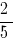and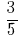Answer: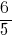Example 2: to Add two fractions with equal denominators:and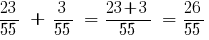Answer: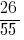### Addition of fractions with different denominators

To add two fractions with different denominators, you need to:

### Examples of addition of fractions with different denominators

Example 3: to Add two fractions with different denominators:and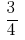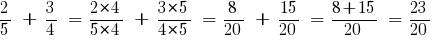Answer:Example 4: to Add two fractions with different denominators:andAnswer: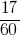To add two mixed numbers, you need to:

• allow fractional part of these numbers to the lowest common denominator;
• separately add the whole parts and fractional parts separately;
• if adding the fractional parts were received improper fraction, to allocate the integer part of this fraction and add it to the obtained integer part;
• reduce the resulting fraction.

### Examples of adding mixed numbers

Example 5: Add two mixed numbers: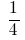and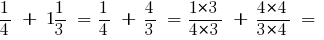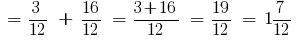Answer: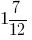## Subtraction of fractions

### Subtraction of fractions with the same denominators

To subtract two fractions with the same denominators, subtract the numerator from the first fraction, the numerator of the second, and the denominator remain unchanged: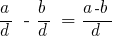### Examples of subtraction of fractions with the same denominators

Example 1: Subtract two fractions with equal denominators: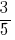and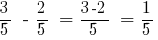Answer: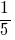### Subtraction of fractions with different denominators

To subtract two fractions with different denominators, you need to:

### Examples of subtracting fractions with different denominators

Example 2: Subtract two fractions with different denominators: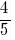and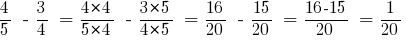Answer: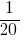### Subtraction of mixed numbers

To subtract two mixed numbers, you need to:

• allow fractional part of these numbers to the lowest common denominator;
• if the fractional part of the minuend is less than the fractional part of the subtractor, to turn it into an improper fraction, reduced by one whole part;
• separately subtract whole parts and fractional parts separately;
• reduce the resulting fraction.

### Examples of subtracting mixed numbers

Example 5: Add two mixed numbers: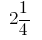andAnswer: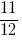## Addition and subtraction of decimal fractions

Addition and subtraction of decimal fractions is performed bitwise. It is convenient to perform in a column.

Detailed information and examples of solving addition and subtracting decimals read here

Tags:
Chapter:
Versions in other languages:
Share with friends: